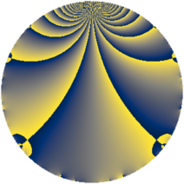# Properties

 Label 507.2.aLevel $507$ Weight $2$ Character orbit 507.a Rep. character $\chi_{507}(1,\cdot)$ Character field $\Q$ Dimension $25$ Newform subspaces $12$ Sturm bound $121$ Trace bound $5$

# Related objects

## Defining parameters

 Level: $$N$$ $$=$$ $$507 = 3 \cdot 13^{2}$$ Weight: $$k$$ $$=$$ $$2$$ Character orbit: $$[\chi]$$ $$=$$ 507.a (trivial) Character field: $$\Q$$ Newform subspaces: $$12$$ Sturm bound: $$121$$ Trace bound: $$5$$ Distinguishing $$T_p$$: $$2$$, $$5$$

## Dimensions

The following table gives the dimensions of various subspaces of $$M_{2}(\Gamma_0(507))$$.

Total New Old
Modular forms 74 25 49
Cusp forms 47 25 22
Eisenstein series 27 0 27

The following table gives the dimensions of the cuspidal new subspaces with specified eigenvalues for the Atkin-Lehner operators and the Fricke involution.

$$3$$$$13$$FrickeDim.
$$+$$$$+$$$$+$$$$6$$
$$+$$$$-$$$$-$$$$7$$
$$-$$$$+$$$$-$$$$9$$
$$-$$$$-$$$$+$$$$3$$
Plus space$$+$$$$9$$
Minus space$$-$$$$16$$

## Trace form

 $$25 q + q^{2} - q^{3} + 27 q^{4} - 2 q^{5} + 3 q^{6} + 4 q^{7} + 9 q^{8} + 25 q^{9} + O(q^{10})$$ $$25 q + q^{2} - q^{3} + 27 q^{4} - 2 q^{5} + 3 q^{6} + 4 q^{7} + 9 q^{8} + 25 q^{9} + 2 q^{10} - 7 q^{12} - 8 q^{14} + 2 q^{15} + 19 q^{16} - 10 q^{17} + q^{18} - 14 q^{20} - 4 q^{21} - 16 q^{22} + 4 q^{23} + 3 q^{24} + 19 q^{25} - q^{27} + 12 q^{28} - 2 q^{29} + 6 q^{30} + 4 q^{31} - 11 q^{32} + 8 q^{33} - 14 q^{34} + 20 q^{35} + 27 q^{36} + 6 q^{37} + 4 q^{38} + 2 q^{40} - 22 q^{41} - 12 q^{42} - 8 q^{43} + 8 q^{44} - 2 q^{45} - 8 q^{46} + 12 q^{47} - 15 q^{48} + 9 q^{49} + 7 q^{50} - 6 q^{51} - 18 q^{53} + 3 q^{54} - 20 q^{55} - 32 q^{56} + 14 q^{58} - 16 q^{59} - 18 q^{60} - 18 q^{61} - 36 q^{62} + 4 q^{63} + 15 q^{64} - 4 q^{66} + 6 q^{68} + 4 q^{69} - 8 q^{70} - 4 q^{71} + 9 q^{72} - 14 q^{73} + 2 q^{74} - 15 q^{75} - 16 q^{76} - 20 q^{79} + 2 q^{80} + 25 q^{81} - 2 q^{82} + 20 q^{84} + 28 q^{85} + 36 q^{86} - 18 q^{87} - 24 q^{88} - 22 q^{89} + 2 q^{90} - 20 q^{92} + 12 q^{93} - 8 q^{94} - 36 q^{95} - q^{96} - 6 q^{97} - 7 q^{98} + O(q^{100})$$

## Decomposition of $$S_{2}^{\mathrm{new}}(\Gamma_0(507))$$ into newform subspaces

Label Dim $A$ Field CM Traces A-L signs $q$-expansion
$a_{2}$ $a_{3}$ $a_{5}$ $a_{7}$ 3 13
507.2.a.a $1$ $4.048$ $$\Q$$ None $$-1$$ $$-1$$ $$-2$$ $$4$$ $+$ $+$ $$q-q^{2}-q^{3}-q^{4}-2q^{5}+q^{6}+4q^{7}+\cdots$$
507.2.a.b $1$ $4.048$ $$\Q$$ None $$-1$$ $$-1$$ $$1$$ $$-2$$ $+$ $+$ $$q-q^{2}-q^{3}-q^{4}+q^{5}+q^{6}-2q^{7}+\cdots$$
507.2.a.c $1$ $4.048$ $$\Q$$ None $$1$$ $$-1$$ $$-1$$ $$2$$ $+$ $+$ $$q+q^{2}-q^{3}-q^{4}-q^{5}-q^{6}+2q^{7}+\cdots$$
507.2.a.d $2$ $4.048$ $$\Q(\sqrt{17})$$ None $$-1$$ $$2$$ $$3$$ $$3$$ $-$ $+$ $$q-\beta q^{2}+q^{3}+(2+\beta )q^{4}+(2-\beta )q^{5}+\cdots$$
507.2.a.e $2$ $4.048$ $$\Q(\sqrt{3})$$ None $$0$$ $$-2$$ $$0$$ $$0$$ $+$ $-$ $$q-q^{3}-2q^{4}+2\beta q^{5}+\beta q^{7}+q^{9}+\cdots$$
507.2.a.f $2$ $4.048$ $$\Q(\sqrt{3})$$ None $$0$$ $$-2$$ $$0$$ $$0$$ $+$ $-$ $$q+\beta q^{2}-q^{3}+q^{4}-\beta q^{6}+2\beta q^{7}+\cdots$$
507.2.a.g $2$ $4.048$ $$\Q(\sqrt{17})$$ None $$1$$ $$2$$ $$-3$$ $$-3$$ $-$ $+$ $$q+\beta q^{2}+q^{3}+(2+\beta )q^{4}+(-2+\beta )q^{5}+\cdots$$
507.2.a.h $2$ $4.048$ $$\Q(\sqrt{2})$$ None $$2$$ $$2$$ $$0$$ $$0$$ $-$ $+$ $$q+(1+\beta )q^{2}+q^{3}+(1+2\beta )q^{4}-2\beta q^{5}+\cdots$$
507.2.a.i $3$ $4.048$ $$\Q(\zeta_{14})^+$$ None $$-3$$ $$-3$$ $$-6$$ $$-2$$ $+$ $+$ $$q+(-1+\beta _{1}+\beta _{2})q^{2}-q^{3}+(4-\beta _{1}+\cdots)q^{4}+\cdots$$
507.2.a.j $3$ $4.048$ $$\Q(\zeta_{14})^+$$ None $$-1$$ $$3$$ $$-4$$ $$-10$$ $-$ $-$ $$q-\beta _{1}q^{2}+q^{3}+\beta _{2}q^{4}+(-2+\beta _{1}+\cdots)q^{5}+\cdots$$
507.2.a.k $3$ $4.048$ $$\Q(\zeta_{14})^+$$ None $$1$$ $$3$$ $$4$$ $$10$$ $-$ $+$ $$q+\beta _{1}q^{2}+q^{3}+\beta _{2}q^{4}+(2-\beta _{1}+\beta _{2})q^{5}+\cdots$$
507.2.a.l $3$ $4.048$ $$\Q(\zeta_{14})^+$$ None $$3$$ $$-3$$ $$6$$ $$2$$ $+$ $-$ $$q+(1-\beta _{1}-\beta _{2})q^{2}-q^{3}+(4-\beta _{1})q^{4}+\cdots$$

## Decomposition of $$S_{2}^{\mathrm{old}}(\Gamma_0(507))$$ into lower level spaces

$$S_{2}^{\mathrm{old}}(\Gamma_0(507)) \cong$$ $$S_{2}^{\mathrm{new}}(\Gamma_0(39))$$$$^{\oplus 2}$$$$\oplus$$$$S_{2}^{\mathrm{new}}(\Gamma_0(169))$$$$^{\oplus 2}$$